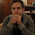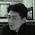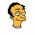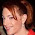27 November 2011

Eleven Equations True Computer Science Geeks Should (at Least Pretend to) Know

This idea is a complete rip off an article that appeared in Wired a little while ago and it got me thinking what would my list for Computer Science look like?  Plus I thought it might be a fun post and unlike the Wired list this one goes to eleven.  So here they are in no particular order:

Binomial Coefficient

The Binomial Coefficient equation generates Pascal’s Triangle and gives you the coefficients for the Binomial Theorem these ideas are often attributed to Pascal but in fact they have been known in part for over a millennia.

As I mentioned that this list is no particular order and I don’t wish to play favorites but if there is one equation here that you should really consider learning and committing to memory this is it. It is central to Combinitorics which has connections to many areas of math, I guess I should qualify that if you are serious about computer science and math related programming topics then you should be able to recite and apply it from memory, even when you are drunk, sleep deprived or being held at gun point. Ok that might be a bit much but you get the idea.  If you are programmer and you haven’t learned it, or need a refresher, I have a post that relates it to the Java Collections API.

Demorgan’s Laws

Logical form:

Set Form:

Ok so that’s like four "equations" for DeMorgan’s Laws, one can’t help but to struck by the similarity between the two sets and this is not a coincidence these two sets of formulas are essentially the same thing, they are special cases of complemented distributive lattices  which means technically it’s really just two formulas:

In this case the ∨ symbol means lattice join operation and the ∧ symbol is the lattice meet operation and the dash with the right downward mark means lattice complementation, I used this to differentiate from the tilde for Boolean complementation.  Also these two formulas are categorical duals of each other, which means that if one were smart and had a good grasp of Category Theory we could probably be really OCD about it and get it down to one formula.

Understanding Set Theory and Boolean Algebra are very important basic concepts in our profession and the Boolean version can make you better programmer, as you can use it to refactor logical if statements

Eigenvector and Eigenvalue

This equation and these concepts are not only central to Linear Algebra but these Linear Algebraic ideas and others are both used and extend into many other areas of math including Linear Transformations and Graphs in terms of matrices like the Adjacency Matrix and much more.

Linear Algebra is becoming increasingly central to the types of things we are doing in our profession, it is used heavily in Statistics and Machine Learning not to mention the Page Rank Algorithm is based in part on this equation.

Pumping Lemma for Regular Languages

No Comp Sci list would be complete with at least one formula from either Formal Language Theory or Automata Theory.  The problem is that these two are pretty different from other areas of math in terms of "Equations" I tried to think of some basic Ideas and The Pumping Lemma came to mind, and I found the above formula concisely expressed on Wikipedia.  This version of the Pumping Lemma is a more limited version of Another Theory which is used to check whether a Language is Regular. Also this is maybe not the best choice as it is pretty dense, if you want a better explanation I recommend this.

While this "equation" is pretty specialized, it is actually a special case of the more general Iteration Theorem, the real point here is really about more general domain knowledge.  It is central to what you do every day you are programming such as compilers and regular expressions not to mention the almost ubiquitous Kleene Star.

Information Entropy

And the formula for Chaitin’s Constant, which is associated with Algorithmic Information Theory and Kolmogorov Complexity:

Interestingly this area has a parallel in the physical word, in terms of Thermodynamic Entropy, which also parallels the Boltzman Equation mentioned in the Wired Article.  Seth Loyd draws some interesting connections between computation and the physical world including Entropy and Boltzman’s Equation.

In programming and computer science information is our business and these ideas are central to many things that we deal with especially the storage, transmission, computational transformation (like compression), and computation of information.  We’ve already seen the possible relationship between both of these theories and Reusability and Software Frameworks.

Bayes' Theorem

Of all of equations on the list, Bayes' Theorem may stand out on its own merits as being one of the most recognizable and it might even qualify as a poster child for the statistical revolution sweeping our industry.  It rose from obscurity to being used for spam detection and with such applications as classifiers, Machine Learning, Data Mining and more.

Mathematically it is part of a rift in statistics and probability and I confess I may not yet call myself "an initiate of the bayesian conspiracy" but it hard to deny its utility plus there seems to be a more axiomatic basis that relates to Boolean Logic Set Theory, which makes it all the more alluring.

Fermat’s Little Theorem

These two equations of Fermat’s Little Theorem are really the same equation written in two different forms, where (a ⊥ p) means coprime and (p ∈ P) means prime.

This is a beautiful little theorem in Number Theory which can be generalized and written even more succinctly using Eulers Totient Function which is represented by φ (n):

These ideas are crucial for understanding encryption algorithms like RSA and there’s this cool result.

Natural Join

Natural Join, like all of the Relational Algebra Join Operations is a composition of the more basic, operations: Selection (σ), Projection (π), Cartesian Product (×), Set Difference (-) and Union (∪).  Natural Join is the Cartesian Product of two tables selecting matching rows and eliminating duplicate columns.  (In the formula above the projection (π) is the set (A) of attributes with duplicates removed and then selects (σ) the set (C) of common attributes which match in both sets and are equal in both sets.)

The Relational Algebra is in my opinion a bit of an odd beast when it comes to algebraic structures, but if you use SQL you are already using an implementation of it.  As data persistence becomes more heterogeneous I think understanding these concepts, will become more important.  Additionally Relational Algebra also has strong ties to Abstract Algebra[ and Category Theory[.

The Fixed-Point (Y) Combinator

Just as we need a "equation" from Formal Language Theory or Automata Theory we really should have one that is related to Lambda Calculus, in this case this equation is in the untyped lambda calculus.  Even though the Fixed-point Combinator stands up on its own, it is fairly well known, well at least in name due to the use of it by Paul Graham for his incubator (Y-Combinator).

The Fixed-Point Combinator allows one to implement anonymous recursion which is a powerful programming technique.  It also ties into some deeper theoretical aspects of computer science and logic such as Combinatory Logic.

O(n)

Many CS majors may cringe at this, and another possible reaction is that’s not much of an equation.  There’s actually quite a bit to this for example did you know there are some substantial and quite beautiful equations associated with this seemingly simple formula.  They are:

Also the following is a definition of (lim sup) Limit Superior:

Where inf is infimum and sup is supremum two concepts that seem to show up quite a bit especially when you are concerned with bounds like with Lattices and they also seem to show up in relation to Topology.

And we all know why we should care about his one: Algorithms, Algorithms, Algorithms.

Euler’s Identity

Euler’s Identity is also listed in Wired’s article and I have to agree that it is one the most beautiful and intriguing formulas in math.

Now this isn’t really as relevant to CS as the other formulas but if you a true computer geek you are hopefully some kind of math geek, and this is such a great formula, not to mention that it appears in The Simpsons' Homer3. It’s actually a specific case (where θ = π) of Euler’s Formula:

These formulas will give one a better understanding complex numbers which are needed in other areas of advanced math also these formulas relate to Fourier Analysis which does have applications in Computer Science.

I am curious to see how well this list holds up, how will I feel about it in a year?  Also in writing this I realized that I still have quite a bit more to learn about at least half of this list, if not all of it, which made it hard to write as it was quite a little (unfinished) research project. I have already written individual articles on several of these equations and have at least two in the works for Linear Algebra, at least two on the Relational Algebra and one planned for Pascal’s Triangle, and those are just the ideas I’ve already had, who knows what the future holds.  I hope you enjoyed it as much as I did writing it.

The Set version may not apply to all cases in set theory, this assumes Union and Intersection over the Power Set of a Set Universe, this would be closed under these operations and would include complements of all elements.  The Set Universe is the upper bound and the empty set is the lower bound.

1.I think a better equation for the Y combinator would be equation that defines it as a fixed-point combinator:

f(Y(f)) = Y(f)

(or, in a more lispy notation,)

(f (Y f)) = (Y f)

Your equation is merely one implementation of a fixed-point combinator, which is not fully general in may ways -- it diverges in a language with applicative-order evaluation, and it is not well-typed in the simply-typed lambda calculus.

2.Perhaps add the logical formulation of induction over the naturals?

P(k) and [\forall n . P(n) => P(n+1)] implies \forall n > k.P(n)

Structural induction tends to be more important in computer science, but the notion of induction is critical.

3.It's interesting to me how little any of these equations have to do with the practical aspects of coding real software. Real life software is dominated by all sorts of graph theory problems, most of which are informal.

4.How can this list be missing Amdahl's law?

5.Interesting post. Must re-read when I can devote my attention to it.

By the way, if you read the post on "Bayes' Conspiracy" on the Coding Horror blog linked to under the Bayes' theorem entry above, and you are still wondering what the correct answer is after all the back and forth in the comments, take a look at:

http://en.wikipedia.org/wiki/Positive_predictive_value

To me, the most fascinating thing about the post is not necessarily the probability (although that's very interesting), it's the fact that those commenters who had the wrong answer were by far the most sure of themselves, and those commenters who figured the problem out correctly were full of doubt. Very interesting sociological experiment.

6.Are these really so important?

I've been coding c++ professionally for 10 years in a field where performance and stability is key and project sizes range from tens of thousands to millions of lines of code.

And I've never ever had to use any of those formulas. (we'll having a feel on what O(N), O(Log N) means, has its advantages, but no idea on the details behind them either).

So where exactly do you need this information? Other than developing algorithms for std/boost or what ever library that 99.9999% of coders use just fine with basic information on performance characteristics.

Correct me if I'm wrong. But I have a feeling that outside standard library development (and scientific / math projects), in real world software projects, these equations are mostly useless information. Wasted hours when one could use the same time learning about design patterns, proper documentation, development process in general, testing and million other things much more worth your time.

(note: English is not my native language so sorry for any typos)

1.While some of the equations mentioned are, even for me, too theoretical (Euler's identity, case in point), keep in mind that theory is the basis of many real-world applications.

Baye's Theorem is the concept behind most, if not all, spam filters. You use De Morgan's Law when optimizing/checking your conditionals. Public-Key Cryptography---a.k.a, why online transactions are secure---wouldn't be possible without concepts like the Binomial Theorem and Fermat's Little Theorem.

I'm not claiming that these theories are the "end all, be all" foundation of today's technology. A spam filter relying solely on Baye's Theorem would be too naive. But making a spam filter without knowledge of Baye's, or at least of probability, is a hopeless effort.

7.great post. thanks for the time and effort for the compilation. also, i must draw attention to this very well laid out online resource for the subject matter: http://MathWorld.Wolfram.com/
all the best.

8.I agree with Karmi. For pure software engineering, these are as useful as knowing the capital of New Zeeland. Things like proper class design, design patterns and comprehensive testing are much more important than the equations above. Unless you're coding some sort of scientific applications where the formulas are actually applied.

9.@Josh, @Karmi, and @Catalin, The title says "computer science geeks", not "corporate hackers". Computer science != programming.

10.Demorgans is is occasionally useful for simplifying complex boolean expressions.

11.Personally, I find that having studied most of the equations on this page, gives me an insight in designing & optimizing algorithms I would not have had otherwise. I strongly disagree with everyone (ie @Karmi, @Catalin) who says I have wasted my time when I could be learning class design. Who says you shouldn't learn both? I have actually applied math theorems when programming games, nothing to do with scientific stuff whatsover.

Regardless, if you can program, you can program, and it would be discriminatory to care how you got to the point of being able to program. If you can be competent without knowing these things, that's fine, but I wouldn't be so quick to dismiss the foundations of math/cs as being irrelevant.

12.hypotenuse^2 = sideA^2 + sideB^2

Yeah, seems pretty elementary, and you might think that everybody knows it off the top of their head. Maybe -- but it's without a doubt the most important and beautiful formula in mathematics, and in this basic form is but the tip of a huge iceberg that comprises a substantial fraction of all mathematics, pure and applied.

13.@luqui yeah sure, Computer science might not equal programming exactly, but I also studied computer science and can only remember O(n) - algorithm complexity - as being significant, the rest of these didn't figure at all. Or maybe it only applies to those at the PhD/Masters level?

14.The formula for O(n) is incorrect. It should be:

f(n) = O(g(n)) iff exists n0, k : forall n > n0 : f(n)/g(n) < k

i.e. for sufficiently large n, f(n) is below a constant factor of g(n).

In your formula, quantification over k is within quantification over n, which makes the formula a tautology.

15.nelhage, I am confused by your last objection. Terms in the simply-typed lambda calculus are strongly normalizing so no implementation of a fixed-point combinator (which can be used to construct divergent terms) can be well-typed in the STLC.

16.I would have recognized DeMorgan's if it used the format I'm more familiar with seeing for negation. A line over the expression.

For a few more of the equations, I was familiar with the concept, but fuck the notation.

For the rest, they seem more likely to be used by someone doing something related to machine learning/AI/information theory than you run of the mill I-spend-all-day-parsing-user-input programmer.

Thank you for making me feel like I'm not a 'true' computer scientist. Next would you like to tell me about how I'm not a 'man' because I don't like to work on cars?

17.Mathematic != PROGAMMING

But if you understand social psychology you can create Internet, which was trully happen .

If you understand mathematic you can do 3d programing and also opmizing code . Mathematic could by used like programmers hammer as also other skils .

18.You've left out the most important formulae that's ubiquitous and always always used by everybody in I.T.:
The Three B's
"Bullshit Baffles Brains"

19.From other point is logic, mathematic and programming the same thing.

And from another point are all things the same.

Then we used to say: University . :)

Steven Jobs learned at the UNIVERSITY kanJi then Apple is happen.

;)

20.Karmi: Did you notice that the equations were for computer science geeks, not for programmers?

21.Josh: If you think that graph theory is mostly informal then there are loads more equations you could do with knowing. You might try reading Bernard Carre's "Graphs and Networks". Graph theory is one of the most formalised areas of computer science.

22."But if you understand social psychology you can create Internet, which was truly happen ."

^This

23.Good article! A fresh reading!

24.Computer scientists are so amusing... do you guys ever get anything done? I've been programming for 30 years and only knew a few of these.

25.@Jasmine... But computer scientists help make complex models to slow down the development process.

Certainly you can see the value in that?

Granted, in the 3D world, if you don't know your computer science, you might as well go home.

26.Ohh man - Y combinator? there are tons of good programmers that have no idea about this ....

27.Formalism tends to dilute learning as far as I know. I was writing a tiny software based 3d engine with texture mapper, and although I knew I could plug in standard homogeneous transformation matrices, i opted to work out the trig on paper and do it manually.
Much more easy to visualize whats happening, for someone reading the code.
A few months ago I "discovered" integral images on my own, only the concept seemed so trivial that I didn't give it a fancy name. Later I find that it's considered a big deal. So I agree that you need to know the math, but conceptual understanding beats the formula any day...

28.What's cool about Euler's is if you rewrite it for pi.

pi=(ln(-1))/(sqrt(-1))

pi in terms of two imaginary numbers.

1.Looking for web references, I put this into Google:

(ln(-1))/(sqrt(-1))

I should have known what would happen. :)

29.It's not much of a generalization, but I prefer the predicate version of De Morgan's:

~∀x p(x) ≡ ∃x ~p(x)
~∃x p(x) ≡ ∀x ~p(x)

30.I am both mathematician and programmer. Unlike many software types, I value knowledge for it's own sake, yet much of it is surprisingly useful anyway.

Unfortunately I can only dream of knowing everything and recognize there must be a limit. But I am still embarrassed by people who are proud of their narrow minds.

31.refreshing]

32.Tommy McGuire: That's interesting, I never thought of the duality between ∀ and ∃ as another formulation of De Morgan's. And I agree that it's another important thing to know.

33.Great post! As a computer scientist and hobby mathematician, I was elated to read this. I am now beginning my fourth year of studies and have come across a few of these (I was so happy to see Fermat's Little Theorem on there because I am in a factorization and primality testing class this semester and a number theory one next).

I think people are getting hung up on the term "computer scientist". I took a class on modern physics and can hold a decently intelligent conversation about Einstein's Theory of Relativity. This in no way makes me a physicists. Just because you program or make computer programs, this does not make you a computer scientist. And if you have a degree in CS, be proud of your background! If you don't like the theory fine, but the importance of the backing theories is relative. Sure you maybe able to write a brute force program to find the shortest path in a graph. But why not search for a better solution? This is a problem I see in out world today. We do what works and hope for the best. We should do what works, but also look for something better.

Ignore the haters man, this was refreshing and a pleasure to read.

34.Your first big-O equivalence is incorrect. The second part of the equivalence you wrote is actually always true, and so your equivalence does not hold if the first part of the equivalence is false (as is if, for instance, we let f(n) = n^2 and g(n) = n). The reason why this is so is because you've put the existential quantifier of k inside the universal quantifier of n, meaning that you've allowed the existence of k to depend on the value of n; but this is always true as we can just let k = 5f(n)/g(n).

The correct way to write it is the other way around; that is, the k existential quantifier must be independent of the universal quantifier of n. In addition, you have to allow for a finite amount of values to not satisfy the inequality utilizing a n0, because big-O talks about asymptotic behavior, not general behavior.

Here's how it should be:

f(n) in O(g(n)) <=> exists n0 in |N: exists k in |R+: forall n in |N: n > n0 => k * g(n) >= f(n).

35.i stay with Euler's identity, Bayes Theorem and the binomial coefficient

36.like dionyziz said, you should change your first big-O equivalence.

37.People who know that mathematics is heart of computer science(CS), will understand the real importance of these equation.

Programming/Coding is just a small aspect of CS. Real CS is mostly about the things which give life to the code written by the developers.

38.You there, this is really good post here. Thanks for taking the time to post such valuable information. Quality content is what always gets the visitors coming. hoverwatch review 2020

39.Thanks for your post. I’ve been thinking about writing a very comparable post over the last couple of weeks, I’ll probably keep it short and sweet and link to this instead if thats cool. Thanks. HELPING PLAN SOFTWARE

40.